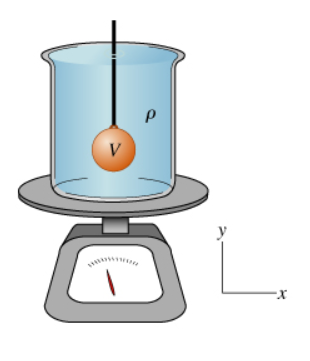# Problem: A cylindrical beaker of height 0.100 m and negligible weight is filled to the brim with a fluid of density ρ = 890 kg/m3. When the beaker is placed on a scale, its weight is measured to be 1.00 N. A ball of density ρb = 5000 kg/m3 and volume V = 60.0 cm3 is then submerged in the fluid so that some of the fluid spills over the side of the beaker. The ball is held in place by a stiff rod of negligible volume and weight. Throughout the problem, assume the acceleration due to gravity is g = 9.81 m/s2A. What is the weight Wb of the ball? Express your answer numerically in newtons.B. What is the reading W2 of the scale when the ball is held in this submerged position? Assume that none of the water that spills over stays on the scale.C. What is the force Fr applied to the ball by the rod? Take upward forces to be positive (e.g., if the force on the ball is downward, your answer should be negative). Calculate your answer from the quantities given in the problem and express it numerically in newtons.D. The rod is now shortened and attached to the bottom of the beaker. The beaker is again filled with fluid, the ball is submerged and attached to the rod, and the beaker with fluid and the submerged ball is placed on the scale. What weight W3 does the scale now show? Express your answer numerically in newtons.

###### FREE Expert Solution

Weight:

$\overline{){\mathbf{w}}{\mathbf{=}}{\mathbf{\rho }}{\mathbf{V}}{\mathbf{g}}}$

A.

Weight, Wb = (5000)(60.0 × 10-6)(9.81) = 2.943 N

The weight Wb of the ball is 2.943 N.

100% (107 ratings)###### Problem Details

A cylindrical beaker of height 0.100 m and negligible weight is filled to the brim with a fluid of density ρ = 890 kg/m3. When the beaker is placed on a scale, its weight is measured to be 1.00 N.

A ball of density ρb = 5000 kg/m3 and volume V = 60.0 cm3 is then submerged in the fluid so that some of the fluid spills over the side of the beaker. The ball is held in place by a stiff rod of negligible volume and weight. Throughout the problem, assume the acceleration due to gravity is g = 9.81 m/s2A. What is the weight Wb of the ball? Express your answer numerically in newtons.

B. What is the reading W2 of the scale when the ball is held in this submerged position? Assume that none of the water that spills over stays on the scale.

C. What is the force Fr applied to the ball by the rod? Take upward forces to be positive (e.g., if the force on the ball is downward, your answer should be negative). Calculate your answer from the quantities given in the problem and express it numerically in newtons.

D. The rod is now shortened and attached to the bottom of the beaker. The beaker is again filled with fluid, the ball is submerged and attached to the rod, and the beaker with fluid and the submerged ball is placed on the scale. What weight W3 does the scale now show? Express your answer numerically in newtons.